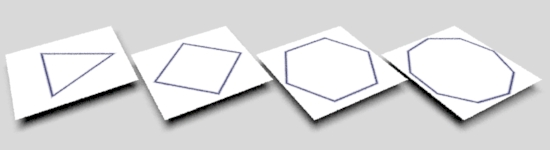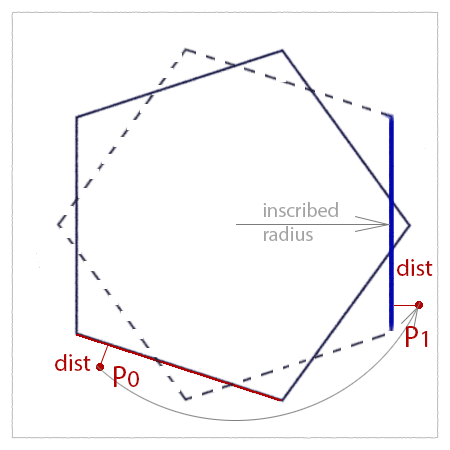### RSLRegular Polygon#### Introduction

This tutorial presents a relatiely simple way of drawing a regular polgon with any number of sides (greater than two). Figure 1 illustrates the approach which is based on the observation that the distance (dist) of a point (P0) to the red edge of the polygon is the same when P0 and the edge are rotated so that the edge is aligned to the vertical axis. If the radius of the inscribed circle of the polygon is known, it is trivial to calculate the distance of P1 to the rotated edge - shown below in blue.

Evaluating the distance based on the x-difference is faster than calculating the distance using a "point-to-line" function - refer to the tutorial "Vectors: Shortest Distance from a Point to a Line". Also, note the length of the polygon side is not taken into account.Figure 1

To calculate angle required to rotate the blue line to the red line we must know in which "segment" the point P0 is located. First, the 'st' texture coordinates of P0 are converted to polar coordinates. In figure 2 assume theta is 125 degrees. Dividing theta by the "interior" angle of 72 degrees gives a ratio of 1.74. Using the floor() function yields a value of 1.0.

Since P is in "segment" 1 an angle of,

1 x 72 + 36 degrees (`segment * interior_angle + half_interior_angle`)

is required to rotate P0 to P1. Assuming the inscribed radius is 0.35 and P1.x of, say 0.37, the distance is,

dist = 0.37 - 0.35 (`abs(inscribed_radius - P1.x)`)Figure 2

If the distance is within an acceptable tolerance the original s,t location (ie. point P0) should receive the foreground color, otherwise, it should ba assigned the background color.

Listing 1 - polygon.sl

 ```surface polygon( float numSides = 4; float innerRad = 0.25; float line = 0.02; color bakcolor = 1; color pencolor = color(0, 0, 0.2) ) { //_____ Convert to polar coordinates______ // Note: positive theta is clockwise. float x = s - 0.5, y = t - 0.5; float R = sqrt(x * x + y * y); float theta = atan(y, x); // -PI to PI radians if(theta < 0.0) theta += 2 * PI; // 0 to 2PI radians //________________________________________ // Find the quadrant and the angle to counter-rotate the // st location so that it's 's' distance from a "vertical" // polygon edge. // Note: positive rotation is anti-clockwise. float interior = (2 * PI)/numSides; float quadrant = floor(theta/interior); float rotation = (quadrant * interior + interior/2); // Because the rotate() function uses 3D points the s,t are // used to define a 3D point (shadingPnt). The axis of rotation // is defined by the center of the polygon and z_axis. point stPnt = point(s - 0.5, 0.5 - t, 0); point origin = point(0, 0, 0); point z_axis = point(0, 0, 1); point rotPnt = rotate(stPnt, rotation, origin, z_axis); // Anti-alias the line float blend = smoothstep(innerRad - line/2, innerRad, rotPnt) * (1 - smoothstep(innerRad, innerRad + line/2, rotPnt)); // Finally, apply the surface color color surfcolor = mix(bakcolor, pencolor, blend); Oi = Os; Ci = Oi * Cs * surfcolor; }```